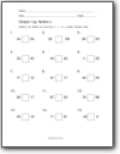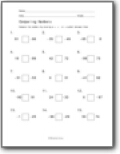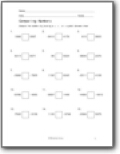# Comparing Numbers Worksheets

#### How Do We Compare Values with Symbols?

Comparing numbers is an important concept, and it's a lot of fun as well. Your child, who started to learn mathematics just now, would find it interesting to learn about different numbers. However, one of the most challenging aspects of comparing two numbers is knowing which symbol to use at what time. Students find it hard to solve them. However, to solve them successfully, we have a trick that can help you solve them. For instance, you have two numbers, 3.5 and 6.7. 3.5 < 6.7. The above inequality shows that 3.5 is less than 6.7. However, if we reversed the numbers, let's see what happens. 6.7 < 3.5.The above inequality shows that 6.7 is greater than 3.5.###### Comparing Numbers 0 to 100

Greater than, less than, or equal. Includes decimals.###### Comparing Numbers 100 to 1000

Students begin to compare values that include decimals and negative numbers.###### Comparing Numbers Four Digits

We work with much larger values than the previous sheet. See what I did there?